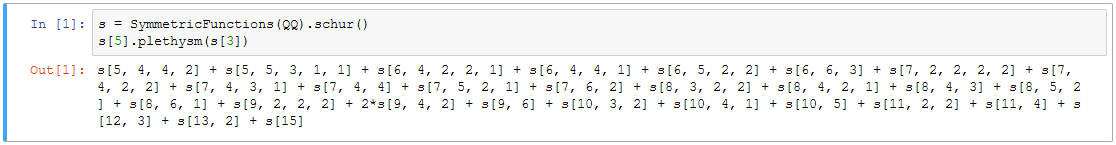# Problem 19

Decompose the space $$\,{\rm Sym}_5({\rm Sym}_3(\mathbb{C}^4))\,$$ of quintic polynomials in the coefficients of a cubic surface in $$\mathbb{P}^3$$ into irreducible $${\rm GL}_4(\mathbb{C})$$-modules.

## Solution

Here is a solution in Sage:

s = SymmetricFunctions(QQ).schur()
s.plethysm(s)


Since the irreducible $${\rm GL}_4(\mathbb{C})$$-modules are indexed by partitions of length at most 4, we can ignore three terms in the above output, and the solution becomes

s[5, 4, 4, 2] + s[6, 4, 4, 1] + s[6, 5, 2, 2] + s[6, 6, 3] + s[7, 4, 2, 2] + s[7, 4, 3, 1] + s[7, 4, 4]
+ s[7, 5, 2, 1] + s[7, 6, 2] + s[8, 3, 2, 2] + s[8, 4, 2, 1] + s[8, 4, 3] + s[8, 5, 2] + s[8, 6, 1]
+ s[9, 2, 2, 2] + 2*s[9, 4, 2] + s[9, 6] + s[10, 3, 2] + s[10, 4, 1] + s[10, 5] + s[11, 2, 2]
+ s[11, 4] + s[12, 3] + s[13, 2] + s.